# Pi Molecular Orbitals of Butadiene

Last updated: January 25th, 2020 |

The Molecular Orbital Diagram of Butadiene, And How To Build It

In the last post in this series we built up the pi molecular orbitals of the allyl pi-system, consisting of three consecutive p orbitals in conjugation. In this article we will show how to build the pi molecular orbital diagram of butadiene.

## 1. Drawing The Pi Molecular Orbitals Of A Conjugated System: A Quick Review

In the last post, we saw that:

• The number of molecular orbitals (n) for a pi-system is equal to the number of contributing p orbitals. For the allyl system, n=3. We had three contributing p orbitals and thus three pi molecular orbitals.
• The lowest-energy orbital always has zero nodes between the p-orbitals (note that we say “no nodes between the p-orbitals” because we’re not counting the node between the individual lobes, which is inherent to all p-orbitals). That is, in the lowest-energy orbital, all phases of the contributing p-orbitals are aligned the same way.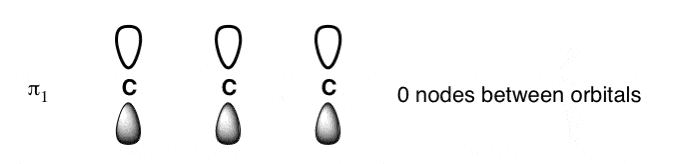• The number of nodes between p-orbitals increases by 1 for each successive energy level, such that the highest-energy orbital has (n-1) nodes (all phases of contributing p-orbitals alternate).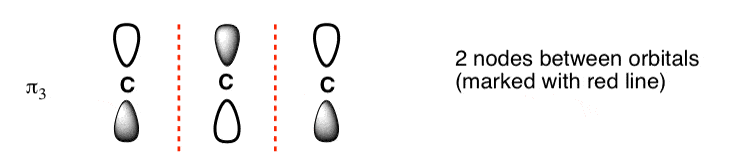• The lowest and highest energy orbitals are always the easiest pi molecular orbitals to draw. It’s helpful to draw them first.
• The trick to drawing the orbital(s) of intermediate energy is to understand where to put the node(s). Nodes are positioned in a way such that they are symmetrical relative to the centre. A system with 1 node has the node smack in the centre (right on the central atom, in fact). A system with 2 nodes will have the nodes at equal distance relative to the centre.
• It’s helpful to draw out all the orbitals of the pi system first, and then add the pi-electrons to it.

Today’s post actually doesn’t introduce any new concepts. We’re just going to take everything from the previous post on building up the orbitals of the allyl system (n=3) and apply it towards drawing the orbitals of the butadienyl system (n=4).

We’ll gain a full appreciation of the importance of the molecular orbitals for butadiene in a little while when we start exploring a curious little reaction discovered by Otto Diels and Kurt Alder back in 1928.

## 2. The Butadienyl Pi System (n = 4)

As the name suggests, butadiene is composed of 4 carbons with two adjacent pi bonds.   As we showed in this earlier post on conjugation and resonance, these two pi bonds are conjugated : all four p orbitals are all aligned with each other, and build up into a larger pi system. It’s for this reason that we can describe the electron density in butadiene with resonance forms, which show alternative distributions of pi electrons in the molecule.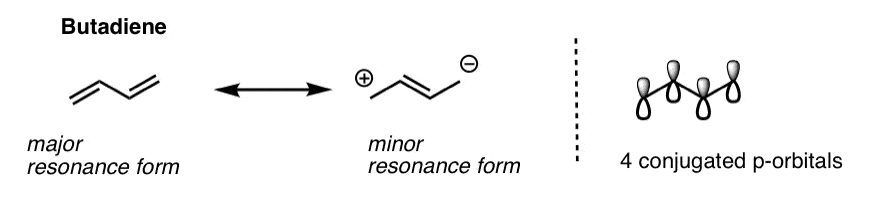Since butadiene consists of 4 individual p orbitals, the pi-system of butadiene will contain 4 pi molecular orbitals.

Using the principles we learned from drawing out the pi molecular orbitals of the allyl system, let’s try to draw out what each of these four molecular orbitals should look like.

## 3. The Lowest-Energy Molecular Orbital (π1) Of The Butadiene Pi System Has Zero Nodes

The lowest energy molecular orbital will have p orbitals with phases in complete alignment with each other. This is very easy to draw: just draw four consecutive p-orbitals with their lobes aligned the same way.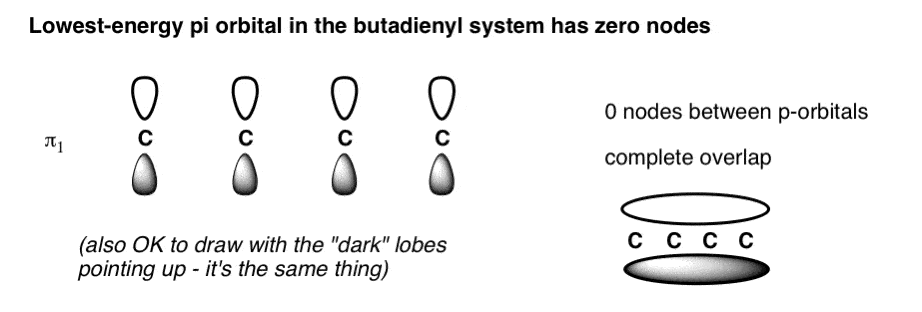We could have also drawn the pi-system with all the shaded lobes pointing up: it’s the same thing. The constructive overlap between the lobes results in a pi orbital that extends over the entire length of the pi system (above right); there are zero nodes between the p orbitals themselves. A physical interpretation of this orbital is that an electron in this orbital is delocalized over the length of the pi system. [note]

## 4. The Highest-Energy Molecular Orbital (π4) Has Three Nodes

The highest-energy molecular orbital is also very easy to draw. Just draw n (4 in our case) p orbitals and alternate the phases of each.  This creates a pi system with three nodes (areas where the lobes change sign). We’ve drawn the nodes in as red dotted lines.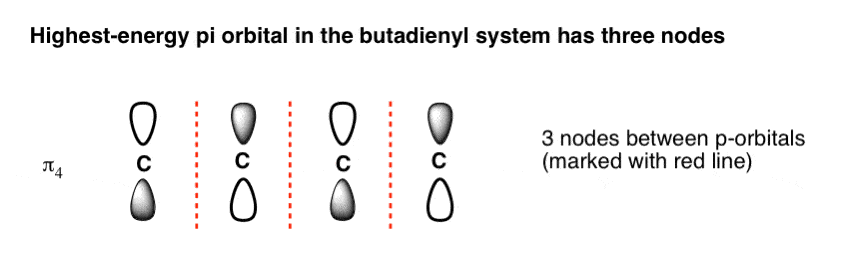## 5. The Second-Lowest-Energy Molecular Orbital (π2) Has One Node

The second-lowest-energy molecular orbital in butadiene will have 1 node. The trick is knowing where to put it.

As we saw with the allyl system, the node cannot just be placed anywhere; the mathematical properties of the Schrödinger wave equation (which we don’t need to get into) dictate its position. Thankfully, node placement for this orbital is pretty straightforward: just plunk it in the middle.(This goes for all systems with a single node, by the way).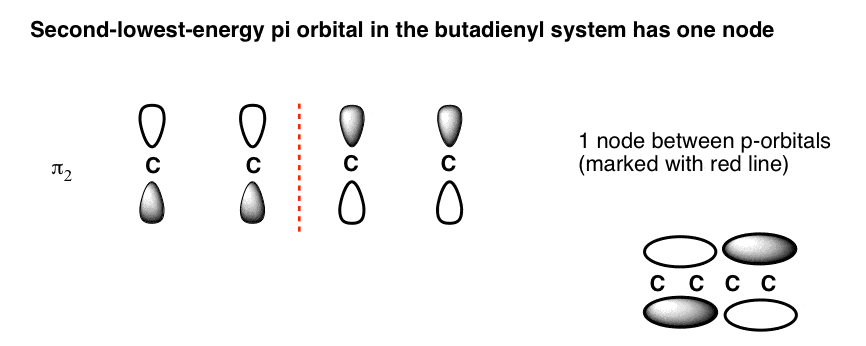Note how the phases flip in the centre of the pi orbital. This corresponds to the situation we’d expect to see for two adjacent non-interacting pi bonds, where the electrons are each confined to pi orbitals spanning two carbons each, with a node in the middle (above right).

## 6. The Third-Lowest-Energy Molecular Orbital (π3) Has Two Nodes

Finally we come to the third-lowest-energy molecular orbital (or second-highest, if you prefer). This has two nodes.

Where to place them?

The general principle is that they are placed symmetrically with respect to the centre.  This is what the orbital picture looks like: [Note]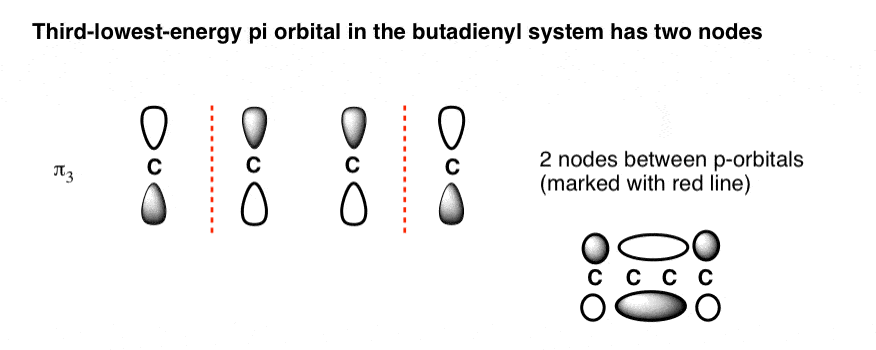This gives us a 2-carbon pi orbital in the centre flanked by two one-carbon orbitals on the sides.

## 7. The Full Molecular Orbital Diagram For The Butadienyl System (n=4)

Now that we have all the pieces, all we need to do to construct the molecular orbital diagram for the butadienyl system is to arrange the orbitals in order of increasing energy. That gives us the following figure (note that we haven’t added any electrons to it yet).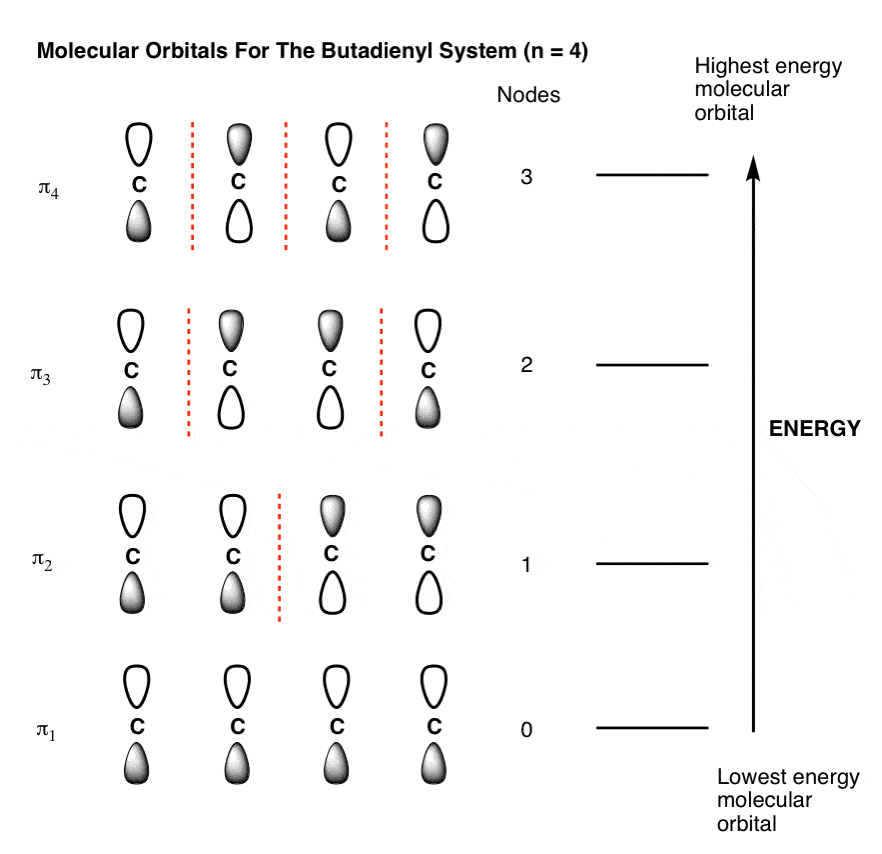A molecular orbital diagram without electrons is like an apartment building without people. So let’s pick the simplest possible molecule to apply to this system: butadiene.

## 8. Populating The Molecular Orbitals Of Butadiene With Electrons

Butadiene has two double bonds with two electrons each, for a total of 4 pi electrons. We fill up the lowest-energy molecular orbitals first, which gives us the following: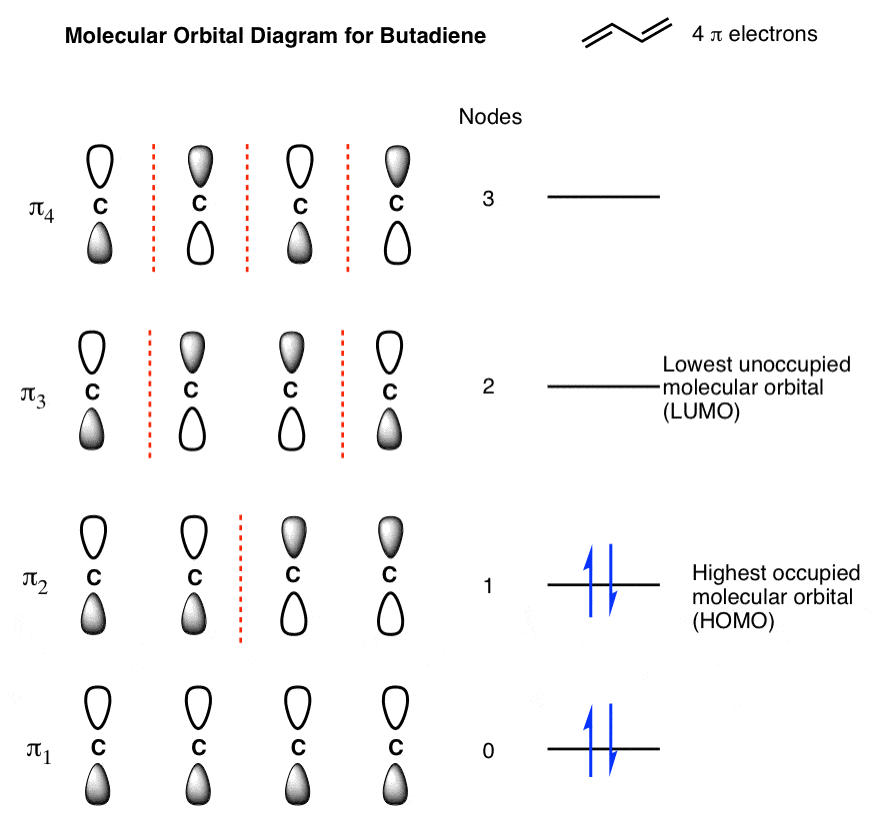We’ve highlighted two molecular orbitals on this diagram as being of particular interest, because, as we’ll see, they’re where the action usually happens. The are sometimes called the “frontier” orbitals.

## 9. Identifying The “HOMO” And “LUMO” Of Butadiene

The highest occupied molecular orbital (HOMO) is π2. You can think of the HOMO as being a little bit like the “valence electrons” of the pi system: they’re the most readily lost. If butadiene participates in a reaction where it is the electron-donor (nucleophile), its electrons are going to come from that orbital.

The lowest-unoccupied molecular orbital (LUMO) is π3. The LUMO is the lowest-energy unoccupied orbital. If butadiene participates in a reaction where it is the electron acceptor (electrophile), the electrons will be donated to that orbital.

We’ll have more to say about the HOMO and LUMO shortly, but that’s sufficient for now.

Now here’s a quiz for you, based on what we’ve gone through today:

• How would you draw the molecular orbital diagram for the pentadienyl cation (n = 5) ?
• Can you draw the MO diagram of the butadienyl radical anion?Thanks to Thomas Struble for assistance with this post.

## Comment section

### 11 thoughts on “Pi Molecular Orbitals of Butadiene”

1. Pingback: CHEMISTRY NOTES
2.Ravi Divakaran says:

Dear James,
Your answers are simple and straight forward, therefore easy to understand. And the questions are very relevant and important for any student of organic chemistry. I love your discussions.

1.James Ashenhurst says:

Hi Ravi, I’m glad you found this post helpful. Thanks for using the site.

3.shuaib ahmed says:

thank you so much your explanations are so fascinating and life saving

thank you a million

1.James Ashenhurst says:

4.Fayeshun Brown says:

Thank you so much! This was very helpful. My professor covered molecular orbitals and determining the HOMO and LUMO so fast! Thanks for such a clear explanation.

1.James Ashenhurst says:

Hi Fayeshun – it does tend to get treated rather quickly, I thought it might be helpful to develop this concept very slowly. If you found it helpful, great!

5.Renuka says:

Thanks for such a great explanation! I have a doubt: in pentadienyl system ,why u placed the two nodes ON carbon 2 and 4 in pi3 orbital.
As you said nodes can be placed at symmetric distance from the central carbon,so nodes should be placed BETWEEN carbon 2-3 and carbon 3-4 ? Why not so?

1.James Ashenhurst says:

Great question. MO calculations, which I’m skipping over here, tell us that any odd-numbered MO in a system of odd-numbered orbitals (e.g. pi3 in the pentadienyl system) will have nodes coinciding with the positions of at least one of the carbons.

6.subha says:

Will it be the same for 1,2-butadiene? bcoz in this molecule only 3 C are involved in pi bonding. The last carbon is not involved in pi bonding. So the total no.of pi orbitals will be 3 ryt?

1.James Ashenhurst says:

No it will not be the same for 1,2-butadiene. If you look at the orbitals in 1,2-butadiene they are at right angles to each other, and are not conjugated. They therefore behave much like two separate pi bonds, not a conjugated system. The analysis in this post does not apply to 1,2-butadiene.
See: https://www.masterorganicchemistry.com/2015/01/13/chiral-allenes-and-chiral-axes/

This site uses Akismet to reduce spam. Learn how your comment data is processed.